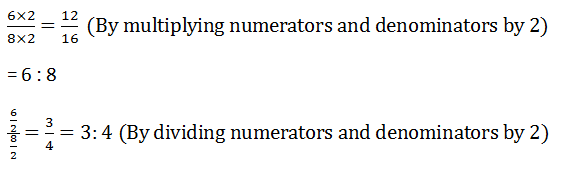# Exercise 9.2

## 1. Which ratio is larger in the following pairs?

### (i) Given 3:4 or 9:16

LCM FOR 4 AND 16 IS 16

3:4 Can be written as = 3/4

3/4 ×(4/4) = 12/16

Clearly 12>9

∴ 3:4 > 9:12

### (ii) Given 15 : 16 or 24 : 25

LCM for 16 and 25 is 400

15 : 16 can be w3ritten as = 15/ 16

15/16×(25/25) = 3375/400

And we have 24/25

24/25 ×(16/1116)  = 384/400

Clearly 384 > 375

∴ 15:16<24 :25

### (iii) Given 4: 7or 5: 8

LCM for 7 and 8 is 56

4: 7 can be written as = 4/7

4/7 ×(8/8) = 32/56

And we have 5/8

5/8 ×(7/7) = 35/56

Clearly 35 > 32

∴ 4: 7 < 5:8

### (iv) Given 9:20 or 8;13

LCM for 20 and 13 is 260

9: 20 can be written as = 9/20

And we have = 8/13

8/13×(20/20) = 160/260

Clearly (20/20) = 160/260

Cleary 160 > 117

∴ 9: 20 < 8:13

### (v) Given 1:2 or 13:27

LCM for 2 and 27 is 54

1:2 Can be written as 1/2

1/2×(27/27) = 13/27

13/27 × (2×2) = 26/54

Clearly 27>26

∴ 1:2 > 13:27

### 2. Give the equivalent ratios of 6: 8.

#### Solution:

Equivalent ratios of 6:8Two equivalent ratios

3:4 = 12:12

### 3. Fill in the following blanks:Updated: December 18, 2019 — 2:59 pm